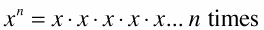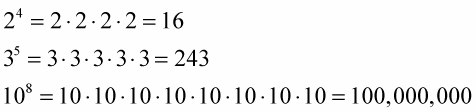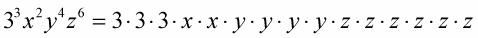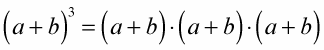##### Basic Math & Pre-Algebra For Dummies Book + Workbook BundleAn exponent is a small, superscripted number written above and to the right of a larger number, the base — this tells you how many times you multiply the base by itself. This repeated multiplication is commonly referred to as the base value being "raised to the power of" the exponential value. Both the base and the exponent can be any real number, and the exponent can be positive or negative, fractional, or even a radical.x, the base, can be any real number.

n, the power, or exponent, can be any real number.

Even though the x in the expression ‘x to the n power’ can be any real number and the n can be any real number, they can’t both be 0 at the same time. ‘0 to the 0 power’ really has no meaning in algebra. It takes a calculus course to discuss this. Also, if x is equal to 0, then n can’t be negative.

In the examples below, the base is multiplied n times, and the exponential expression is evaluated.The nice thing about exponents of ten is that the power tells you how many zeros are in the answer.

In this example, several bases are multiplied together. Each base has its own, separate exponent. The x, y, and z represent real numbers.You can see why it’s preferable to use the powers. And in the next example, the base is actually a binomial. The parentheses mean that you add the two values together before applying the exponent.